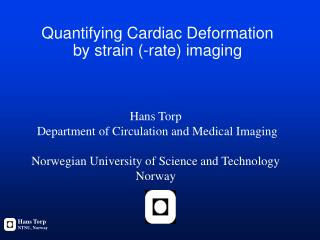DownloadDownload PresentationQuantifying Cardiac Deformation by strain (-rate) imaging

# Quantifying Cardiac Deformation by strain (-rate) imaging

Télécharger la présentation## Quantifying Cardiac Deformation by strain (-rate) imaging

- - - - - - - - - - - - - - - - - - - - - - - - - - - E N D - - - - - - - - - - - - - - - - - - - - - - - - - - -
##### Presentation Transcript

1. Hans Torp NTNU, Norway Quantifying Cardiac Deformation by strain (-rate) imaging Hans Torp Department of Circulation and Medical Imaging Norwegian University of Science and Technology Norway

2. Hans Torp NTNU, Norway Quantifying Cardiac Deformation by strain (-rate) imaging • Describe deformation by strain and strain rate • Ultrasound methods for strain rate • speckle tracking versus Doppler methods • Clutter noise and thermal noise • angle dependency • Frame rate issues • Visualization of strain and strain-rate

3. Velocity gradient – strain rate V2 – V1 L Velocity gradient: = Rate of deformation = Strain Rate V2 L V1 (Myocardial) velocity gradient is an instantaneous property

4. Integrated velocity gradientversus strain ”Growth function” Strain = exp{ IVG } - 1

6. RF Envelope

7. What is the best velocity estimator? velocity ~ angle(R) R=x1*x2 s2 x2 s1 x1 Autocorrelation method is optimal (Maximum likelihood estimator) RF signals IQ signals

8. What is the best velocity estimator? v=c/4piT angle(R) R=x1*x2

9. Hans Torp NTNU, Norway Estimation error is minimumwhen correlation is maximum Velocity estimate Correlation magnitude

10. Linear regression Weighted Linear regression (Maximun likelihood) Estimate of velocity gradient (strain rate) 0.03 0.025 0.02 Velocity [m/sec] 0.015 0.01 0 0.005 0.01 0.015 depth range [mm]

11. Simulation experimentStrain rate estimators Linear regression Strain rate Weighted Linear regression (Maximun likelihood) Simulation no

12. Clutter noise

13. Clutter noise • bias towards zero for velocity measurements • increased variance for strain rate • Clutter filter helps when tissue velocity is high • limited effect in apical region • Second harmonic (octave) imaging reduces clutter • independent of tissue velocity

14. 2. harmonic Hans Torp NTNU, Norway Fundamental and second harmonic signal separated by filter 50 100 150 200 250 300 350 400 450 20 40 60 80 100 Fundamental Signal from septum Noise from LV cavity

15. Second Harmonic TDI Fundamental, f=1.67MHz • Fundamental and second harmonic calculated from the same data set • No significant noise difference • Second harmonic TDI gives more aliasing. Second harmonic, f=3.33MHz

16. Fundamental, f=1.67MHz Second harmonic, f=3.33MHz Second Harmonic SRI • Fundamental and second harmonic calculated from the same data set • Significant noise reduction when using the second harmonic frequency band • Aliasing is not a problem due to small velocity differences

17. Frame rate issues in tissue velocity and strain rate imaging Packet acquisition tissue interleaving 100 - 150 frames/sec Continuous acquisition tissue interleaving 250 - 350 frames/sec - TVI aliasing + Offline spectral Doppler (Work in progress) Packet acquisition 30 - 80 frames/sec Image sector: 70 deg. Parallell beams : 2

18. Packet acquisition - continuous acquisition

19. v1 v2 Hans Tarp NTNU, Norway Myocardial velocity and strain ratewith 300 frames/sec Velocity Strain rate Time

20. Lateral movement

21. Angle corrected strain

22. Summary 1 • Strain rate from Tissue Doppler is possible for motion along the ultrasound beam with high temporal resolution • Weighted linear regression gives minimum estimation error • Second harmonic Tissue Doppler reduce clutter noise artefacts in strain rate imaging

23. Summary 2 • Integrated strain is improved by tracking material points • 2D speckle-tracking gives angle-independent strain, with reduced temporal resolution • A combination of high frame rate tissue Doppler and lower frame rate speckle tracking is probably the best solution for strain imaging • 3D reconstruction of strain (-rate) covering the left ventricle can be obtained from 3 standard apical views